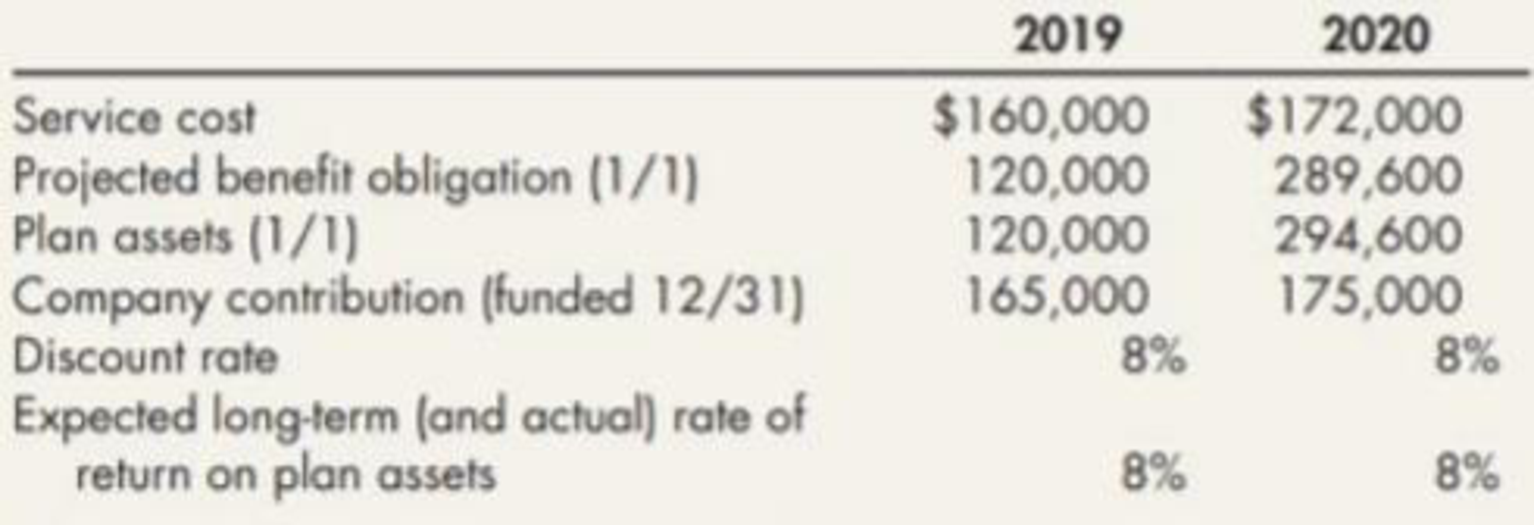Chapter 19, Problem 5E### Intermediate Accounting: Reporting...

3rd Edition
James M. Wahlen + 2 others
ISBN: 9781337788281

#### Solutions

Chapter
Section### Intermediate Accounting: Reporting...

3rd Edition
James M. Wahlen + 2 others
ISBN: 9781337788281
Textbook Problem
1 views

# Baron Company adopted a defined benefit pensionplan on January 1, 2018. The following information pertains to the pension plan for 2019 and 2020:There are no other components of Baron’s pension expense.Required: 1. Compute the amount of Baron's pension expense for 2019 and 2020. 2. Prepare the journal entries to record the pension expense for 2019 and 2020.

1.

To determine

Calculate the amount of pension expense of Company B for 2019 and 2020.

Explanation

Pension plan: Pension plan is the plan devised by corporations to pay the employees an income after their retirement, in the form of pension.

Calculate the amount of pension expense of Company B for 2019:

 Particulars 2019 2020 Amounts in ($) Amounts in ($) Service cost $160,000$172,000 Add: Interest cost $9,600 (1)$23,168(2) Less: Expected return on plan assets $9,600 (1)$23,568 (2) Pension expense for 2019 $160,000$171,600

Table (1)

Working note (1):

Calculate the interest cost for 2019:

Interest cost = Projected benefit obligtion×Discount rate=$120,000×8100=$9,600

Calculate the interest cost for 2020:

2.

To determine

Prepare the necessary journal entries of Company B to record the pension expense for 2019 and 2020.

### Still sussing out bartleby?

Check out a sample textbook solution.

See a sample solution

#### The Solution to Your Study Problems

Bartleby provides explanations to thousands of textbook problems written by our experts, many with advanced degrees!

Get Started